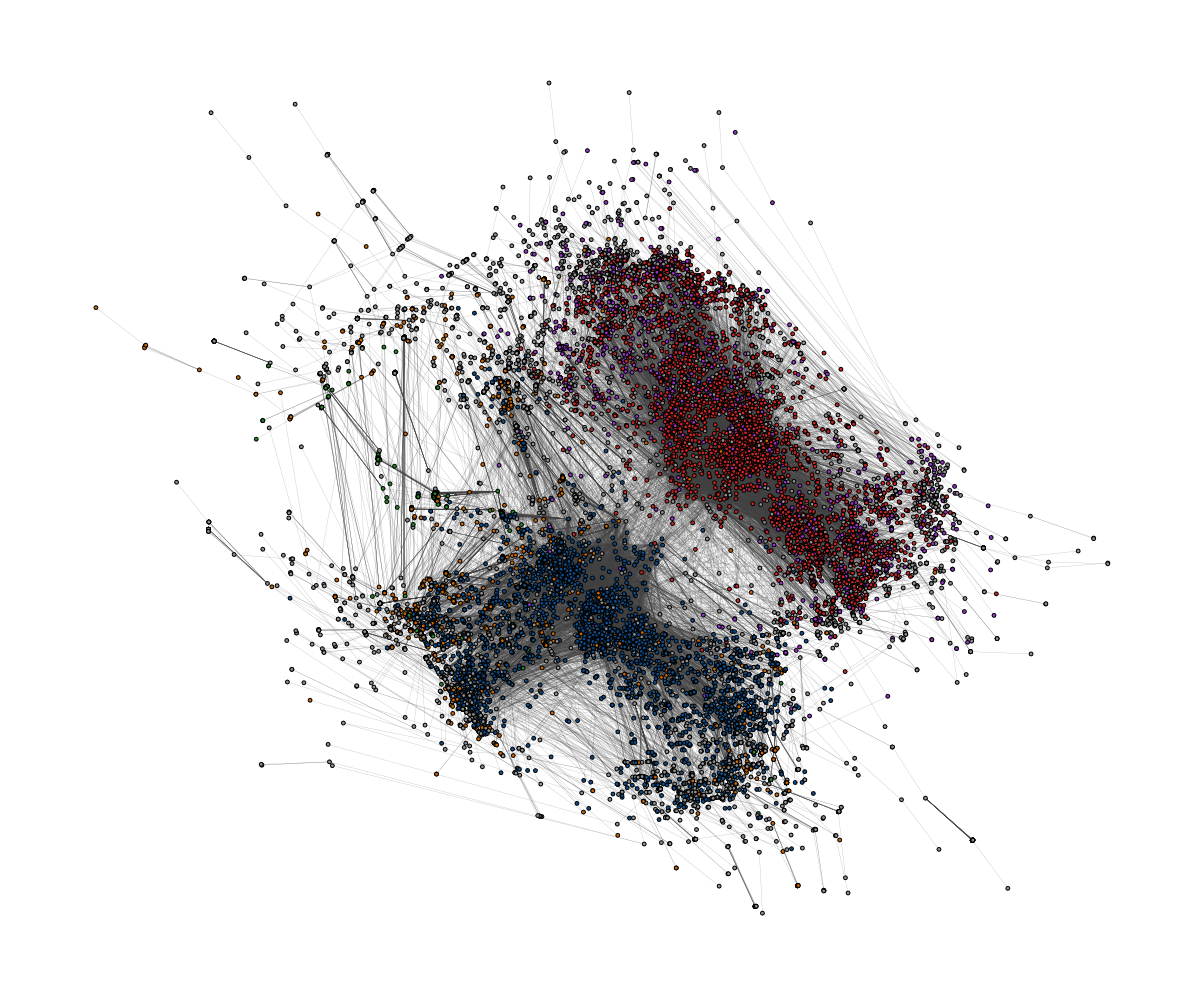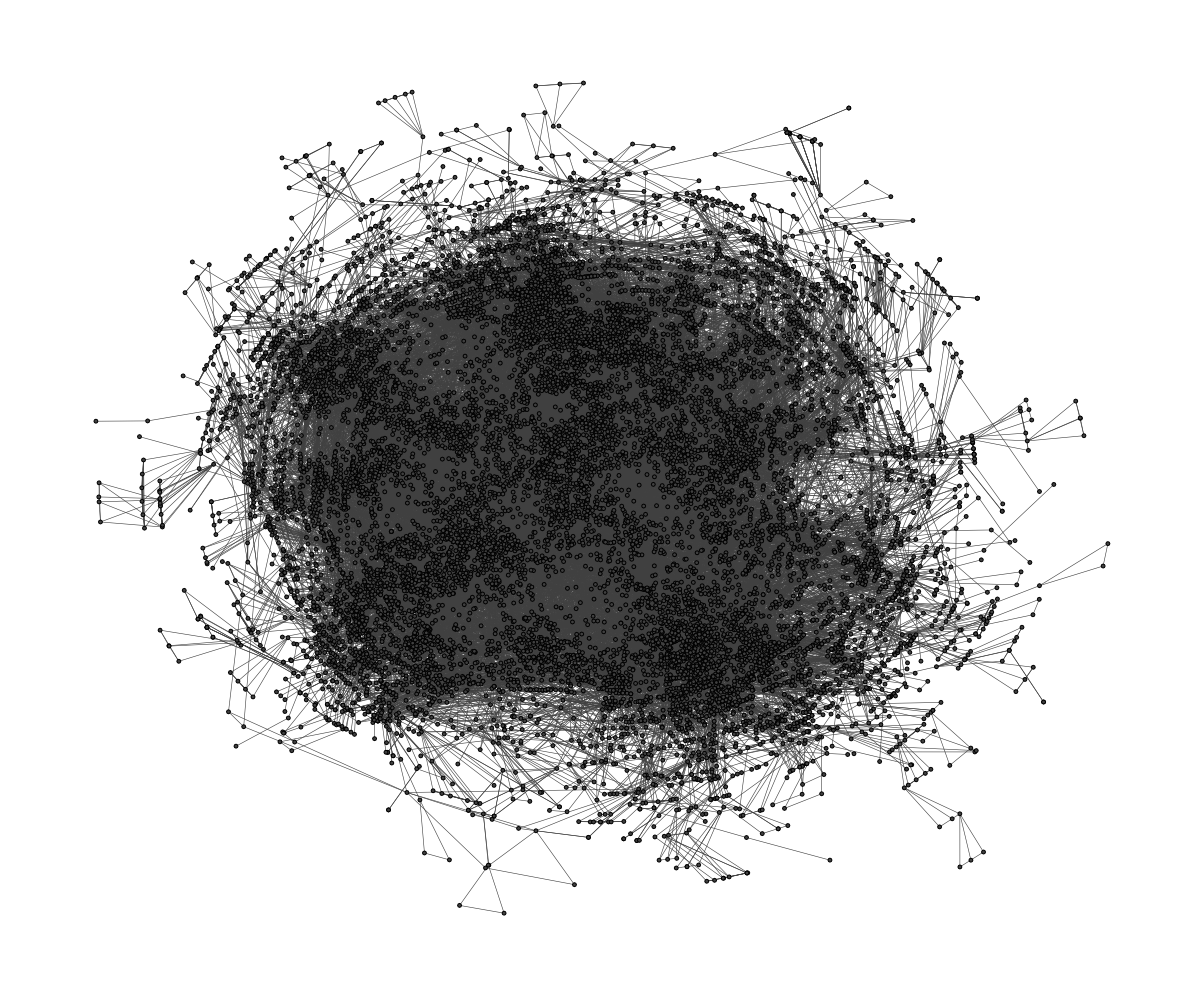# graphlayouts

An R package for network visualization

9/12/18

The package implements the following algorithms:

• Stress majorization (Paper)
• sparse stress (Paper)
• pivot MDS (Paper)
• dynamic layout for longitudinal data (Paper)
• a simple multilevel layout

## Install

``````# dev version
remotes::install_github("schochastics/graphlayouts")

#CRAN
install.packages("graphlayouts")``````

## Stress Majorization

This example is a bit of a special case since it exploits some weird issues in igraph.

``````library(igraph)
library(ggraph)
library(graphlayouts)

set.seed(666)
pa <- sample_pa(1000,1,1,directed = F)

ggraph(pa,layout = "nicely")+
geom_node_point(col="black",size=0.3)+
theme_graph()``````
``````
ggraph(pa,layout="stress")+
geom_node_point(col="black",size=0.3)+
theme_graph()``````

Stress majorization also works for networks with several components. It relies on a bin packing algorithm to efficiently put the components in a rectangle, rather than a circle.

``````set.seed(666)
g <- disjoint_union(
sample_pa(10,directed = F),
sample_pa(20,directed = F),
sample_pa(30,directed = F),
sample_pa(40,directed = F),
sample_pa(50,directed = F),
sample_pa(60,directed = F),
sample_pa(80,directed = F)
)

ggraph(g,layout = "nicely") +
geom_node_point() +
theme_graph()``````
``````
ggraph(g, layout = "stress",bbox = 40) +
geom_node_point() +
theme_graph()``````

## Backbone Layout

Backbone layouts are helpful for drawing hairballs.

``````set.seed(665)
#create network with a group structure
g <- sample_islands(9,40,0.4,15)
g <- simplify(g)
V(g)\$grp <- as.character(rep(1:9,each=40))

ggraph(g,layout = "stress")+
geom_node_point(aes(col=grp))+
scale_color_brewer(palette = "Set1")+
theme_graph()+
theme(legend.position = "none")``````

The backbone layout helps to uncover potential group structures based on edge embeddedness and puts more emphasis on this structure in the layout.

``````bb <- layout_as_backbone(g,keep=0.4)
E(g)\$col <- F
E(g)\$col[bb\$backbone] <- T

ggraph(g,layout="manual",x=bb\$xy[,1],y=bb\$xy[,2])+
geom_node_point(aes(col=grp))+
scale_color_brewer(palette = "Set1")+
scale_edge_color_manual(values=c(rgb(0,0,0,0.3),rgb(0,0,0,1)))+
theme_graph()+
theme(legend.position = "none")``````

## Radial Layout with Focal Node

The function `layout_with_focus()` creates a radial layout around a focal node. All nodes with the same distance from the focal node are on the same circle.

``````library(igraphdata)
library(patchwork)
data("karate")

p1 <- ggraph(karate,layout = "focus",focus = 1) +
draw_circle(use = "focus",max.circle = 3)+
geom_node_point(aes(fill=as.factor(Faction)),size=2,shape=21)+
theme_graph()+
theme(legend.position = "none")+
coord_fixed()+
labs(title= "Focus on Mr. Hi")

p2 <- ggraph(karate,layout = "focus",focus = 34) +
draw_circle(use = "focus",max.circle = 4)+
geom_node_point(aes(fill=as.factor(Faction)),size=2,shape=21)+
theme_graph()+
theme(legend.position = "none")+
coord_fixed()+
labs(title= "Focus on John A.")

p1+p2``````

The function `layout_with_centrality` creates a radial layout around the node with the highest centrality value. The further outside a node is, the more peripheral it is.

``````library(igraphdata)
library(patchwork)
data("karate")

bc <- betweenness(karate)
p1 <- ggraph(karate,layout = "centrality", centrality = bc, tseq = seq(0,1,0.15)) +
draw_circle(use = "cent") +
annotate_circle(bc,format="",pos="bottom") +
geom_node_point(aes(fill=as.factor(Faction)),size=2,shape=21)+
theme_graph()+
theme(legend.position = "none")+
coord_fixed()+
labs(title="betweenness centrality")

cc <- closeness(karate)
p2 <- ggraph(karate,layout = "centrality", centrality = cc, tseq = seq(0,1,0.2)) +
draw_circle(use = "cent") +
annotate_circle(cc,format="scientific",pos="bottom") +
geom_node_point(aes(fill=as.factor(Faction)),size=2,shape=21)+
theme_graph()+
theme(legend.position = "none")+
coord_fixed()+
labs(title="closeness centrality")

p1+p2``````

## Large graphs

`graphlayouts` implements two algorithms for visualizing large networks (<100k nodes). `layout_with_pmds()` is similar to `layout_with_mds()` but performs the multidimensional scaling only with a small number of pivot nodes. Usually, 50-100 are enough to obtain similar results to the full MDS.

`layout_with_sparse_stress()` performs stress majorization only with a small number of pivots (~50-100). The runtime performance is inferior to pivotMDS but the quality is far superior.

A comparison of runtimes and layout quality can be found in the wiki
tl;dr: both layout algorithms appear to be faster than the fastest igraph algorithm `layout_with_drl()`.

Below are two examples of layouts generated for large graphs using `layout_with_sparse_stress()`A retweet network with 18k nodes and 61k edgesA co-citation network with 12k nodes and 68k edges